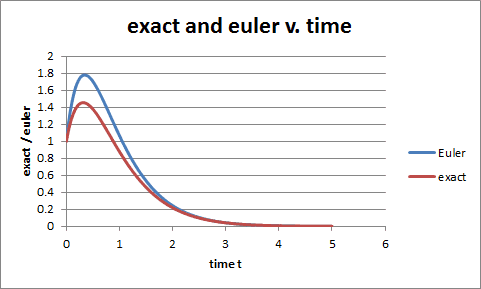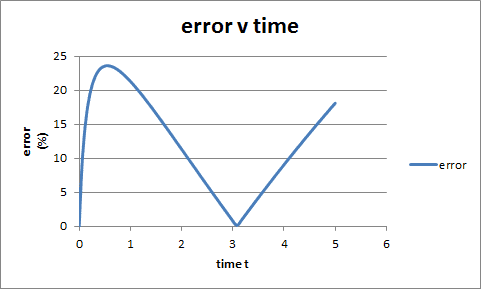# Euler's Method to approximate a second order Differential Equation

• Yosty22
Homework Statement y'' + 4y' + 4y = 0 ---- y(0) = 1, y'(0) = 5Find the exact solution of the differential equation.Use the exact solution and Euler's Method to compute Euler's Approximation for time t = 0 to t = 5 using a step h=0.05. Plot Euler's & Exact vs. t and plot Error vs. t. Then, answer the following question:Is the error really increasing? That is, is Euler's method becoming less accurate as t increases?Homework Equationsx1 = yx2 = y'x1' = y'x2' = y'' = -4

## Homework Statement

y'' + 4y' + 4y = 0 ---- y(0) = 1, y'(0) = 5
Find the exact solution of the differential equation.
Use the exact solution and Euler's Method to compute Euler's Approximation for time t = 0 to t = 5 using a step h=0.05. Plot Euler's & Exact vs. t and plot Error vs. t. Then, answer the following question:

Is the error really increasing? That is, is Euler's method becoming less accurate as t increases?

## Homework Equations

x1 = y
x2 = y'
x1' = y'
x2' = y'' = -4y' - 4y = -4x2 - 41
Euler's Method Equations assuming the substitutions made above:
x1(n+1) = x1(n) + hx/2(n)
x2(n+1) = x2(n) + h*(y''(n))

## The Attempt at a Solution

[/B]
First, I solved the differential equation. Writing the characteristic equation r2 + 4r + 4 = 0, I solved for there to be a repeated root at r = -2. Therefore, the general solution is:
y= c1e-2t + c2te-2t
Taking a derivative to use the initial conditions:
y' = -c1/2 * e-2t + c2te-2t/2 + c2e-2t
Applying the initla conditions, I find c1 = 1 and c2 = 11/2
Therefore, the solution becomes:
e-2t + 11t/2 * e-2t

I then created an excel spreadsheet which calculate and then plots the exact solution vs. the eulerapproximation, giving me the graph:then the error graph looks as follows:So around time t = 3, it makes sense that the error would be about 0 due to how Euler approximations work. Since Euler Approximations use the tangent lines of the functions at a certain point to approximate the shape of the graph, the more and more linear the solution gets (such as from t --> infinity in this solution), the easier it is to approximate the solution because the slope of a horizontal line is 0. However, I have absolutely no idea what could be going on here that would cause the error to start increasing seemingly linearly after time t = 3 where the solution and the euler approximation is practically horizontal. I cannot fathom that a repeated root would cause this error. Does anyone have any idea what could be causing this?

Sorry, I thought I could be more a little more explicit about my response at the bottom. Since Euler Approximations go off of the slope of the tangent line of the exact solution and as t is increasing, the slope of the tangent line of the solution approaches 0, so the error should be approaching 0. So to answer the question, I would say that the error isn't really increasing despite what the graph displays. However, I'm not sure how to answer the part of the question asking to explicitly state what would cause it to appear as if it was increasing.

Yosty22 said:

## Homework Statement

y'' + 4y' + 4y = 0 ---- y(0) = 1, y'(0) = 5
Find the exact solution of the differential equation.
Use the exact solution and Euler's Method to compute Euler's Approximation for time t = 0 to t = 5 using a step h=0.05. Plot Euler's & Exact vs. t and plot Error vs. t. Then, answer the following question:

Is the error really increasing? That is, is Euler's method becoming less accurate as t increases?

## Homework Equations

x1 = y
x2 = y'
x1' = y'
x2' = y'' = -4y' - 4y = -4x2 - 41
Euler's Method Equations assuming the substitutions made above:
x1(n+1) = x1(n) + hx/2(n)
x2(n+1) = x2(n) + h*(y''(n))

## The Attempt at a Solution

[/B]
First, I solved the differential equation. Writing the characteristic equation r2 + 4r + 4 = 0, I solved for there to be a repeated root at r = -2. Therefore, the general solution is:
y= c1e-2t + c2te-2t
Taking a derivative to use the initial conditions:
y' = -c1/2 * e-2t + c2te-2t/2 + c2e-2t

The derivative of $e^{kt}$ is $ke^{kt}$, not $\frac1k e^{kt}$.

Yosty22 said:

## Homework Statement

y'' + 4y' + 4y = 0 ---- y(0) = 1, y'(0) = 5
Find the exact solution of the differential equation.
Use the exact solution and Euler's Method to compute Euler's Approximation for time t = 0 to t = 5 using a step h=0.05. Plot Euler's & Exact vs. t and plot Error vs. t. Then, answer the following question:

Is the error really increasing? That is, is Euler's method becoming less accurate as t increases?

## Homework Equations

x1 = y
x2 = y'
x1' = y'
x2' = y'' = -4y' - 4y = -4x2 - 41
Euler's Method Equations assuming the substitutions made above:
x1(n+1) = x1(n) + hx/2(n)
x2(n+1) = x2(n) + h*(y''(n))

## The Attempt at a Solution

[/B]
First, I solved the differential equation. Writing the characteristic equation r2 + 4r + 4 = 0, I solved for there to be a repeated root at r = -2. Therefore, the general solution is:
y= c1e-2t + c2te-2t
Taking a derivative to use the initial conditions:
y' = -c1/2 * e-2t + c2te-2t/2 + c2e-2t
Applying the initla conditions, I find c1 = 1 and c2 = 11/2
Therefore, the solution becomes:
e-2t + 11t/2 * e-2t

I then created an excel spreadsheet which calculate and then plots the exact solution vs. the eulerapproximation, giving me the graph: View attachment 74110
then the error graph looks as follows:
View attachment 74111
So around time t = 3, it makes sense that the error would be about 0 due to how Euler approximations work. Since Euler Approximations use the tangent lines of the functions at a certain point to approximate the shape of the graph, the more and more linear the solution gets (such as from t --> infinity in this solution), the easier it is to approximate the solution because the slope of a horizontal line is 0. However, I have absolutely no idea what could be going on here that would cause the error to start increasing seemingly linearly after time t = 3 where the solution and the euler approximation is practically horizontal. I cannot fathom that a repeated root would cause this error. Does anyone have any idea what could be causing this?